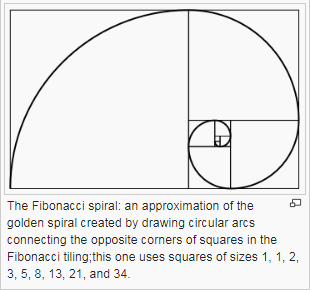A B C D E
F G H I J
K L M N O
P Q R S T
U V X Y Z

## Fibonacci and Metatronic ReversalIn mathematics, the Fibonacci numbers or Fibonacci sequence are the numbers in the following integer sequence: 1,1,2,3,5,8,13,21,34,55,89,144...

If we sum the squares of any series of Fibonacci numbers, they will equal the last Fibonacci number used in the series times the next Fibonacci number. This property results in the Fibonacci spiral, based on the following progression and properties of the Fibonacci series.The Fibonacci spiral gets closer and closer to a Golden Spiral as it increases in size because of the ratio of each number in the Fibonacci series to the one before it converges on Phi, 1.618, as the series progresses. Beginning with Zero, then 1, it then moves on to the next number, as such : 0+1=1, 1+1=2, 2+1=3, 3+2=5, 5+3=8 and so forth.

When we apply this mathematical formula to quantify or measure the movement of energy or Consciousness within time or space, the Fibonacci Spiral loses its connection going back to the Zero point or Source, instead the sequence uses the previous number to add into itself to get to the next higher number of the sequence. The Fibonacci Spiral illustrates the math used to perpetuate the war over energy, therefore the consciousness suppression on the earth, as when the sequence grows in number or size, it is due to the consumption of the previous values in order to grow itself larger. The larger it gets, the more it progressively moves out and away from the Krystal Spiral of the original core manifestation body or divine template body, as it expands. Therefore the Fibonacci Spiral is a model of Metatronic Reversal in the consciousness fields that ultimately attach like a parasite to a living host, collecting waste products, such as accumulating toxic Miasma fields which form into the Qlippoth or Adverse Sephiroth, in the Phantom Matrix to infect the Universal Tree of Life.

The Fibonacci spiral sequence emerges from a point of attachment, which is a parasitic in action, to attach itself to a living form, consuming everything in its path, to which it progressively loses contact when it continually expands itself. It grows and expands its consciousness through the consumption and eventual annihilation of that which it had attached itself to and had existed previously. This is what quantifies the actions of a Fallen race or species, such as the Black Suns Regressive or Belial groups.

References: www.energeticsynthesis.com